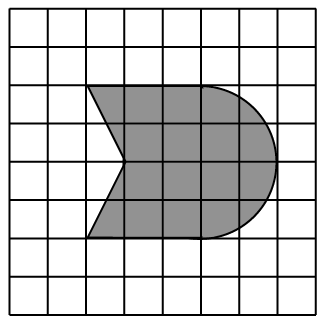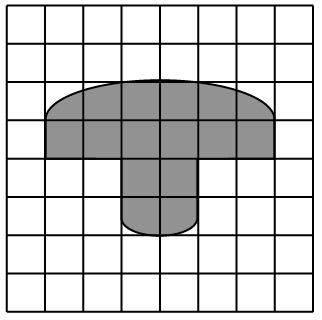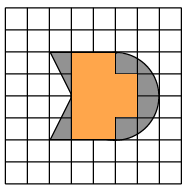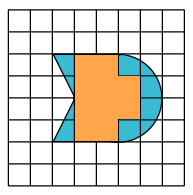### Home > CCG > Chapter 1 > Lesson 1.2.2 > Problem1-66

1-66.

Bertie placed a transparent grid made up of unit squares over each of the shapes she was measuring below.  Using her grid, approximate the area of each region.

1.1.Approximate the area by counting the squares. Count squares completely filled as one unit squared, and those only partially filled as one-half a unit squared.$10$$10$

$10(1\text{ unit})+10\left ( \frac{1}{2} \text{ units}\right)=15\text{ units}^2$